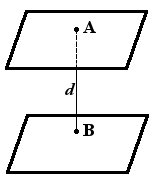Study of mathematics online.
Study math with us and make sure that "Mathematics is easy!"

# Online calculator. Distance between two planes

This online calculator will help you to find distance between two planes.

Using this online calculator, you will receive a detailed step-by-step solution to your problem, which will help you understand the algorithm how to find distance between two planes.

## Distance between two planes calculatorFirst plane equation:

x + y + z + = 0

Second plane equation:

x + y + z + = 0

You can input only integer numbers, decimals or fractions in this online calculator (-2.4, 5/7, ...). More in-depth information read at these rules.